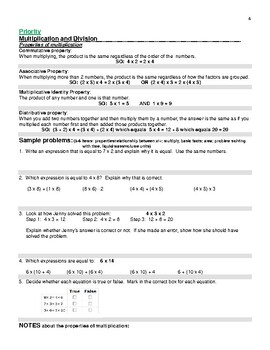# Grade 3 Math Student Study Guide and Sample ProblemsSubject
Resource Type
File Type

PDF

(1 MB|47 pages)
Standards
• Product Description
• Standards

You can print out copies of this document for each student to use. Everything needed is contained within it. There are "short review sections" for each of the grade-level standards, as assessed on SBA Summative. This revision includes Blueprints for the SBA Summative, IABs, Student Data Tracker, and summarized information for accessing the IAB and ICA data within CAASPP, for CA teachers.

There are sample problems, parallel in format to the SBA Summative, that students, in pairs, can work through to give them practice in solving real-life application problems.

The standards/targets are aligned with the CA Math Standards and the Common Core Standards for grade 3.

Teachers could also provide students with the information found in this document, so that they can add it to their own interactive math journals during the year.

Recognize area as additive. Find areas of rectilinear figures by decomposing them into non-overlapping rectangles and adding the areas of the non-overlapping parts, applying this technique to solve real world problems.
Use tiling to show in a concrete case that the area of a rectangle with whole-number side lengths 𝘢 and 𝘣 + 𝘤 is the sum of 𝘢 × 𝘣 and 𝘢 × 𝘤. Use area models to represent the distributive property in mathematical reasoning.
Partition shapes into parts with equal areas. Express the area of each part as a unit fraction of the whole. For example, partition a shape into 4 parts with equal area, and describe the area of each part as 1/4 of the area of the shape.
Understand that shapes in different categories (e.g., rhombuses, rectangles, and others) may share attributes (e.g., having four sides), and that the shared attributes can define a larger category (e.g., quadrilaterals). Recognize rhombuses, rectangles, and squares as examples of quadrilaterals, and draw examples of quadrilaterals that do not belong to any of these subcategories.
Compare two fractions with the same numerator or the same denominator by reasoning about their size. Recognize that comparisons are valid only when the two fractions refer to the same whole. Record the results of comparisons with the symbols >, =, or <, and justify the conclusions, e.g., by using a visual fraction model.
Total Pages
47 pages
Included
Teaching Duration
1 Year
Report this Resource to TpT
Reported resources will be reviewed by our team. Report this resource to let us know if this resource violates TpT’s content guidelines.